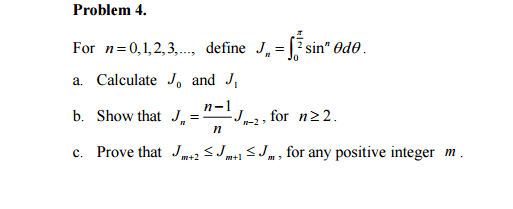# Reduction formula (sinx)^n inequality

• kenok1216

## Homework Statementpart c

## The Attempt at a Solution

Jm+2=m+2-1/m+2 Jm=m+1/m+2 Jm
hence Jm+2<Jm
should i expend Jm+2 Jm+1 Jm to the term J0 then compare them?
why the inequality is <= but not <?
should i use M.I to proof it??[/B]

Last edited:
kenok1216 said:

## Homework Statement

View attachment 102041
part c

## The Attempt at a Solution

Jm+2=m+2-1/m+2 Jm=m+1/m+2 Jm
hence Jm+2<Jm
should i expend Jm+2 Jm+1 Jm to the term J0 then compare them?
why the inequality is <= but not <?
should i use M.I to proof it??[/B]

Please stop using a bold font; it looks like you are yelling at us.

$$J_{m+2} = m+2 - \frac{1}{m} + 2J_m = m + \frac{1}{m} + 2J_m$$
Use the basic property of integrals that if $f(x) \geq g(x)$ for all $x \in (a,b)$ then $\int_a^b f(x)\,dx \geq \int_a^b g(x)\,dx$.